# MCQ in Differential Calculus (Limits and Derivatives) Part 1 | ECE Board Exam

(Last Updated On: February 5, 2020)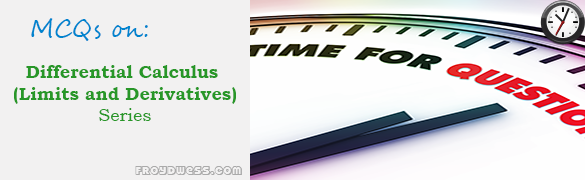This is the Multiple Choice Questions Part 1 of the Series in Differential Calculus (Limits and Derivatives) topic in Engineering Mathematics. In Preparation for the ECE Board Exam make sure to expose yourself and familiarize in each and every questions compiled here taken from various sources including but not limited to past Board Examination Questions in Engineering Mathematics, Mathematics Books, Journals and other Mathematics References.

### Multiple Choice Questions Topic Outline

• MCQs in Derivatives | MCQs in Derivatives of Algebraic functions | MCQs in Derivatives of Exponential functions | MCQs in Derivatives of Logarithmic functions | MCQs in Derivatives of Trigonometric functions | MCQs in Derivatives of Inverse Trigonometric functions | MCQs in Derivatives of Hyperbolic functions

### Start Practice Exam Test Questions Part I of the Series

Choose the letter of the best answer in each questions.

Problem 1: CE Board November 1997

Evaluate the Limit: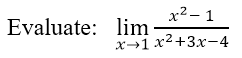• A. 1/5
• B. 2/5
• C. 3/5
• D. 4/5

Problem 2: ECE Board April 1998

Evaluate the Limit: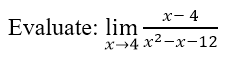• A. undefined
• B. 0
• C. Infinity
• D. 1/7

Problem 3: ME Board April 1998

Evaluate the Limit: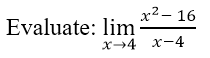• A. 0
• B. 1
• C. 8
• D. 16

Problem 4: ECE Board April 1993

Evaluate the Limit: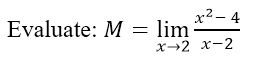• A. 0
• B. 2
• C. 4
• D. 6

Problem 5: EE Board April 1995

Evaluate the Limit: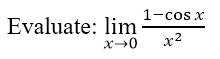• A. 0
• B. 1/2
• C. 2
• D. -1/2

Problem 6: ME Board October 1997

Compute the following limit: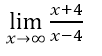• A. 1
• B. 0
• C. 2
• D. Infinite

Problem 7: EE Board October 1994

Evaluate the Limit: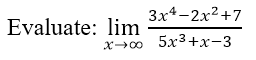• A. Undefined
• B. 3/5
• C. Infinity
• D. Zero

Problem 8: ECE Board November 1991

Evaluate the Limit: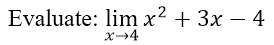• A. 24
• B. 26
• C. 28
• D. 30

Problem 9: ECE Board November 1994

Evaluate the Limit: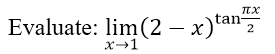• A. e2π
• B. e2/π
• C. 0
• D. α

Problem 10: EE Board October 1997

Differentiate y = ex cos x2

• A. –ex sin x2
• B. ex (cos x2 – 2x sin x2)
• C. ex cos x2 – 2x sin x2
• D. -2xex sin x

Problem 11: EE Board October 1997

Differentiate y = sec (x2 + 2)

• A. 2x cos (x2 + 2)
• B. –cos (x2 + 2) cot (x2 + 2)
• C. 2x sec (x2 + 2) tan (x2 + 2)
• D. cos (x2 +2)

Problem 12: CE Board October 1994

What is the derivative with respect to x of (x + 1)3 – x3?

• A. 3x + 6
• B. 3x – 3
• C. 6x – 3
• D. 6x + 3

Problem 13: EE Board October 1997

Differentiate y = log10 (x2 + 1)2

• A. 4x (x2 + 1)
• B. (4x log10 e) / (x2 + 1)
• C. log e(x) (x2 + 1)
• D. 2x (x2 + 1)

Problem 14: EE Board October 1997

Differentiate (x2 + 2)1/2

• A. ((x2 + 2)1/2) / 2
• B. x / (x2 + 2)1/2
• C. (2x) / (x2 + 2)1/2
• D. (x2 + 2)3/2

Problem 15: EE Board October 1997

If y = (t2 + 2)2 and t = x1/2, determine dy/dx

• A. 3/2
• B. (2×2 + 2x) / 3
• C. 2(x + 2)
• D. x5/2 + x1/2

Problem 16: ME Board April 1997

What is the first derivative of the expression (xy)x = e?

• A. 0
• B. x/y
• C. –y [(1 + ln xy) / x)]
• D. –y [(1 – ln xy) / x2)]

Problem 17: ME Board April 1998

Find the derivative with respect to x function √(2 – 3×2)

• A. (-2×2) / √(2 – 3×2)
• B. (-3x) / √(2 – 3×2)
• C. (-3×2) / √(2 – 3×2)
• D. (3x) / √(2 – 3×2)

Problem 18: EE Board April 1995

Find y’ if y = arcsin cos x

• A. -1
• B. -2
• C. 1
• D. 2

Problem 19: CE Board May 1997

Find the derivative of arccos 4x.

• A. -4 / (1 – 16×2)0.5
• B. 4 / (1 – 16×2)0.5
• C. -4 / (1 – 4×2)0.5
• D. 4 / (1 – 4×2)0.5

Problem 20: CE Board November 1996

Find the derivative of (x + 1)3 / x

• A. ((x + 1)2 / x) – ((x + 1)3 / x)
• B. (4(x + 1)2 / x) – (2(x + 1)3 / x)
• C. (2(x + 1)3 / x) – ((x + 1)3 / x3)
• D. (3(x + 1)2 / x) – ((x + 1)3 / x2)

Problem 21: ECE Board November 1991

Differentiate the equation y = x2 / (x +1)

• A. (x2 + 2x) / (x + 1)2
• B. x / (x + 1)
• C. 2x
• D. (2×2) / (x + 1)

Problem 22: CE Board November 1995

The derivative with respect to x of 2cos2 (x2 + 2) is

• A. 2sin (x2 + 2) cos (x2 + 2)
• B. -2sin (x2 + 2) cos (x2 + 2)
• C. 8x sin (x2 + 2) cos (x2 + 2)
• D. -8x sin (x2 + 2) cos (x2 + 2)

Problem 23: CE Board November 1993

Find the second derivative of y by implicit differentiation from the equation 4×2 + 8y2 = 36

• A. 64×2
• B. (– 9/4) y3
• C. 32xy
• D. (- 16/9) y3

Problem 24: ME Board April 1998

Find the partial derivative with respect to x of the function xy2 – 5y + 6.

• A. y2 – 5
• B. y2
• C. xy – 5y
• D. 2xy

Problem 25: ME Board October 1997

Find the second derivative of x3 – 5×2 + x = 0

• A. 10x – 5
• B. 6x – 10
• C. 3x + 10
• D. 3×2 – 5x

Problem 26: ME Board April 1998

Given the function f(x) = x to the 3rd power – 6x + 2. Find the first derivative at x = 2.

• A. 6
• B. 7
• C. 3×2 – 5
• D. 8

Problem 27: CE Board May 1996

Find the slope of the ellipse x2 + 4y2 – 10x – 16y + 5 = 0 at the point where y = 2 + 80.5 and x = 7.

• A. -0.1463
• B. -0.1538
• C. -0.1654
• D. -0.1768

Problem 28: EE Board October 1997

If y = 4cos x + sin 2x, what is the slope of the curve when x = 2 radians?

• A. -2.21
• B. -4.94
• C. -3.95
• D. 2.21

Problem 29: ECE Board November 1991

Find the slope of the line tangent to the curve y = x3 – 2x + 1 at x = 1.

• A. 1
• B. 1/2
• C. 1/3
• D. 1/4

Problem 30: ECE Board November 1991

Given the slope of the curve at the point (1, 1): y = (x3/4) – 2x + 1

• A. 1/4
• B. -1/4
• C. 1 1/4
• D. -1 1/4

Problem 31: ECE Board November 1998

Find the slope of x2y = 8 at the point (2, 2)

• A. 2
• B. -1
• C. -1/2
• D. -2

Problem 32: CE Board May 1998

Find the slope of the curve x2 + y2 – 6x + 10y + 5 + 0 at point (1, 0).

• A. 1/5
• B. 2/5
• C. 1/4
• D. 2

Problem 33: CE Board May 1996

Find the slope of the tangent to the curve, y = 2x – x2 + x3 at (0, 2).

• A. 1
• B. 2
• C. 3
• D. 4

Problem 34: ECE Board April 1999

Find the coordinates of the vertex of the parabola y = x2 – 4x + 1 by making use of the fact that at the vertex, the slope of the tangent is zero.

• A. (2, -3)
• B. (3, -2)
• C. (-1, -3)
• D. (-2, -3)

Problem 35: ECE Board April 1999

Find the equation of the normal to x2 + y2 = 5 at the point (2, 1)

• A. y = 2x
• B. x = 2y
• C. 2x + 3y = 3
• D. x + y = 1

Problem 36: CE Board May 1995

What is the equation of the normal to the curve x2 + y2 = 25 at (4, 3)?

• A. 5x + 3y = 0
• B. 3x – 4y = 0
• C. 3x + 4y = 0
• D. 5x – 3y = 0

Problem 37: EE Board April 1997

Locate the points of inflection of the curve y = f(x) = x2 ex.

• A. -2 ± √3
• B. 2 ± √2
• C. -2 ± √2
• D. 2 ± √3

Problem 38: ECE Board November 1991

In the curve 2 + 12x – x3, find the critical points.

• A. (2, 18) and (-2, -14)
• B. (2, 18) and (2, -14)
• C. (-2, 18) and (2, -14)
• D. (-2, 18) and (-2, 14)

Problem 39: CE Board November 1997

Find the radius of curvature of a parabola y2 – 4x = 0 at point (4, 4).

• A. 22.36 units
• B. 25.78 units
• C. 20.33 units
• D. 15.42 units

Problem 40: ECE Board November 1996

Find the radius of curvature at any point in the curve y + ln cos x = 0.

• A. cos x
• B. 1.5707
• C. sec x
• D. 1

### Online Questions and Answers in Differential Calculus (Limits and Derivatives) Series

Following is the list of multiple choice questions in this brand new series:

Differential Calculus (Limits and Derivatives) MCQs
PART 1: MCQs from Number 1 – 50                        Answer key: PART I
PART 2: MCQs from Number 51 – 100                   Answer key: PART II

P inoyBIX educates thousands of reviewers and students a day in preparation for their board examinations. Also provides professionals with materials for their lectures and practice exams. Help me go forward with the same spirit.

“Will you subscribe today via YOUTUBE?”

Subscribe

#### One Response

1.S.Deepanjali Nayak
PinoyBIX Engineering. © 2014-2020 All Rights Reserved | How to Donate? |